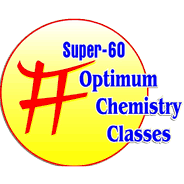# What is pseudo order reaction? Give one example of it

## Pseudo Order Reaction Define

The reaction which appears to be higher order kinetic but actually follow lower order kinetic is called Pseudo order reaction.

We can understand the concept of pseudo order reaction with the help of following example :-

Ex : CH_3 COOC_2 H_5+H_2 O → CH_3 〖COOH+C〗_2 H_5 OH

Experimentally found that –

Rate =K[CH_3 COOC_2 H_5 ][H_2 O]

[K×[H_2 O]=K^′]

Rate =K^′  [CH_3 COOC_2 H_5]

Order of reaction =1

## COLLISION THEORY OR CONCEPT OF ACTIVATION ENERGY

According to collision theory reactant molecules collide with each other to form product but all types of collision are not responsible for the formation of product only fraction of collision are responsible for the formation of product.

Ø

### There are two types of collision on the basis of collision theory :-

1. Effective
2. Ineffective

(i)EFFECTIVE :- Such type of collision which are responsible the formation of product is called effective collision.

(ii)INEFFECTIVE :- Such type of collision which are not responsible for the formation of product is called ineffective collision.

## THRESHOLD ENERGY

1. The minimum energy posesed by reactant molecules for effective collision is called threshold energy.
2. It is denoted by E_T.

## ACTIVATION ENERGY

1. The additional energy which must be observed by reactant molecules so that they achieve threshold energy or energy or energy barrier is called activation energy.
2. It is denoted by E_a.
3. The value of activation energy is always positive.
4. Activation energy = Threshold energy-Enthalpy of reactant

E_a=E_T-E_R

Where,

E_P= Enthalpy of product

E_R= Enthalpy of reactant

E_T= Threshold energy

E_a= Activation energy  of reactant.

E_a^′= Activation energy  of product

vFor Exothermic –

Note

1. E_R>E_P

2. E_a<E_a^′

3. ∆H=-ve